Function Repository Resource:

# PeriodicPatternGenerator

Generate a periodic pattern

Contributed by: Ed Pegg Jr
 ResourceFunction["PeriodicPatternGenerator"][{n,{x,y},disp}] creates a periodic square array with a side length of x×y based on repeated x×y rectangles filled with the digits of integer n in base 2 with displacement disp on subsequent rows. ResourceFunction["PeriodicPatternGenerator"][{n,{x,y},disp,b}] uses base b.

## Details

Periodic patterns can be equivalent by toroidal displacement. For example, an 8×8 checkerboard pattern with a black square in the lower right corner is equivalent to an 8×8 checkerboard pattern with a white square in the lower right corner after a toroidal displacement of {a,b} for any odd a+b.
For the counts below, an n-ary pattern is assumed to have n colors.
Up to order 16, there are 0, 3, 8, 20, 36, 112, 144, 452, 740, 1824, 2232, 9848, 8820, 28302, 54015, 129064 distinct binary patterns.
Up to order 10, there are 0, 0, 8, 65, 180, 1123, 2064, 11391, 27187, 104774 distinct ternary patterns.

## Examples

### Basic Examples (5)

Create a periodic pattern based on the binary expansion of 3::

 In:=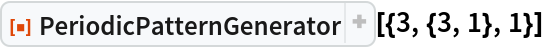Out=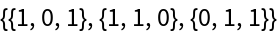Show a binary periodic pattern:

 In:=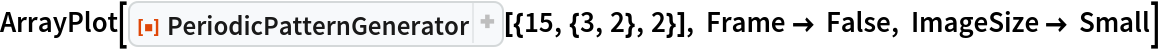Out=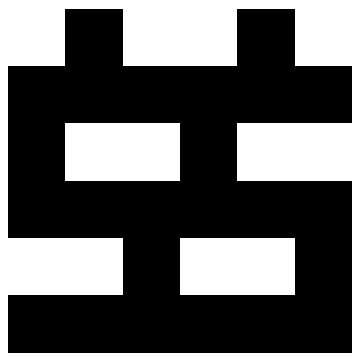Show the order 2 binary periodic patterns (horizontal stripes, vertical stripes, checkerboard):

 In:=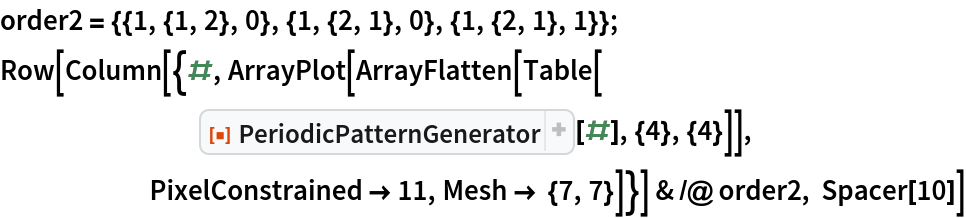Out=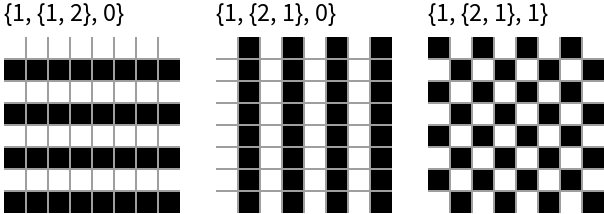Show all eight order 3 binary periodic patterns:

 In:=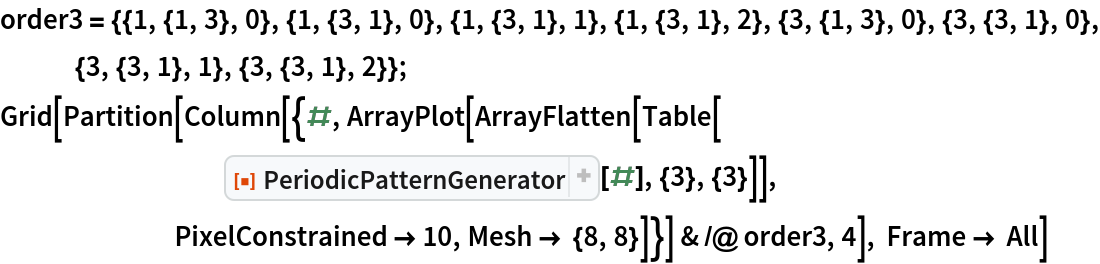Out=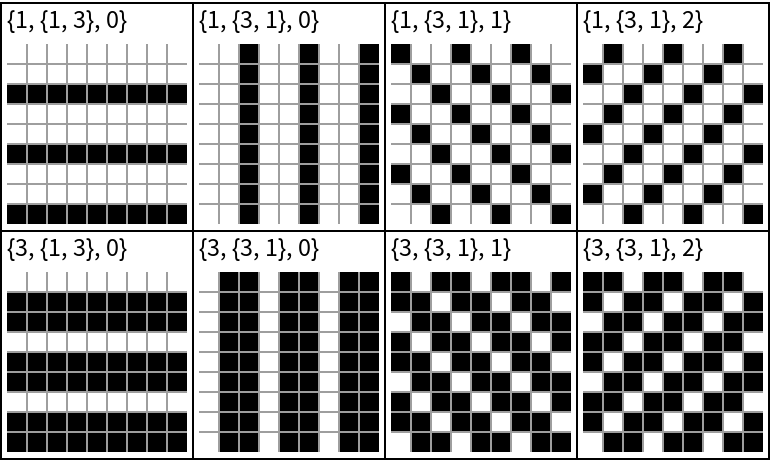Show all twenty order 4 periodic patterns (with Tooltips):

 In:=Out=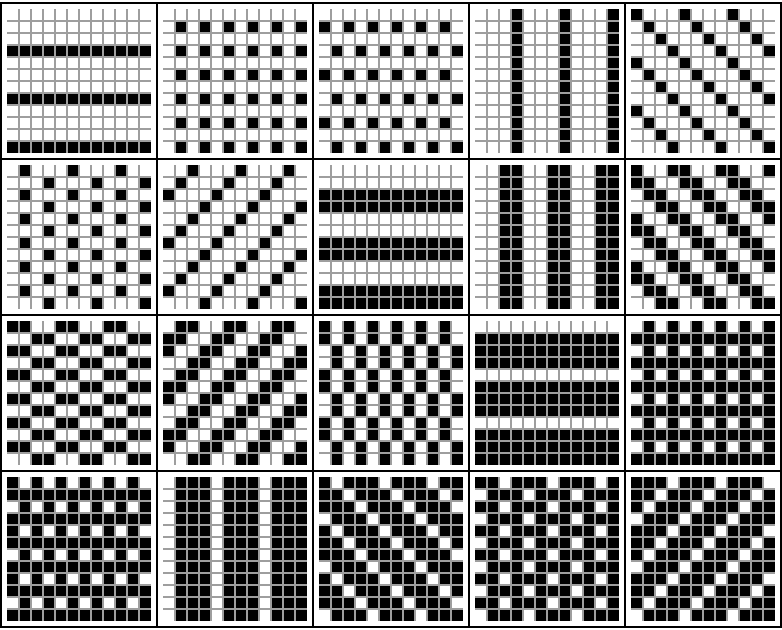### Scope (1)

These periodic patterns are equivalent by displacement:

 In:=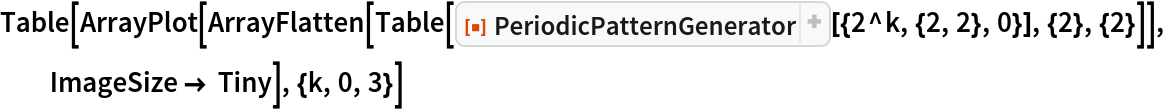Out=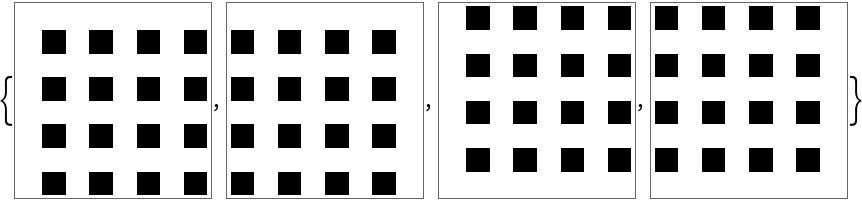### Options (2)

There are 8 order-3 ternary periodic patterns:

 In:=Out=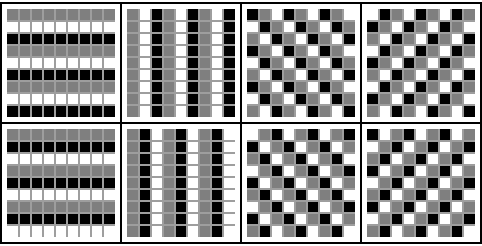There are 65 order-4 ternary periodic patterns:

 In:=Out=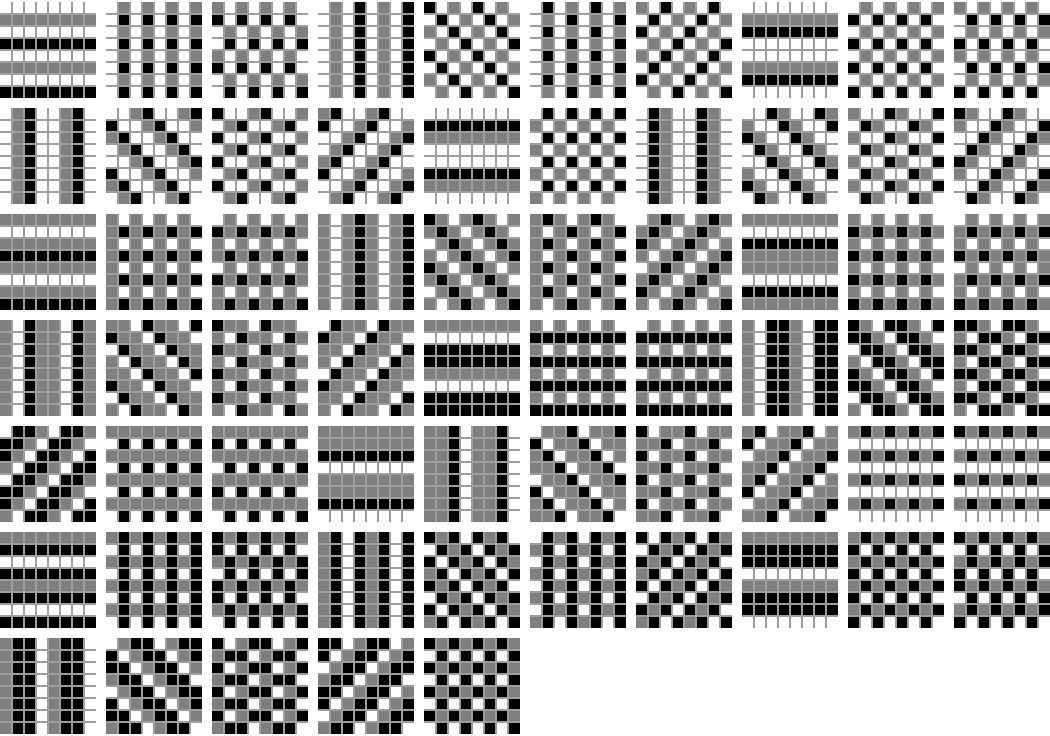### Possible Issues (1)

Boring patterns usually have a simpler representation:

 In:=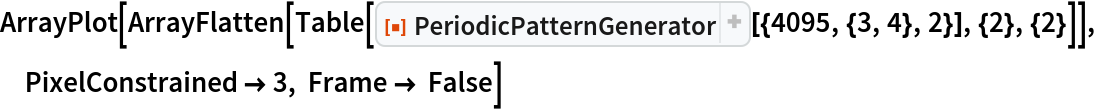Out=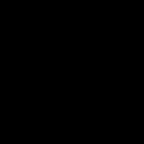In:=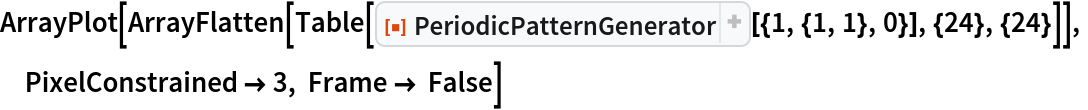Out=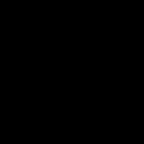### Neat Examples (3)

Using PeriodicPatternGenerator, CanonicalListRotation and a brute force algorithm, we can find all displacement-distinct binary patterns up to order 9, given here as a convenience:

 In:=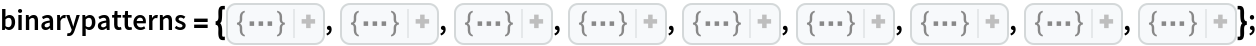Show all 180 order 5 ternary patterns:

 In:=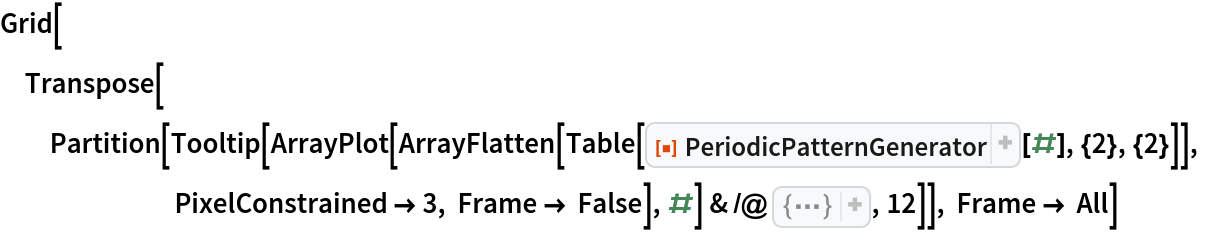Out=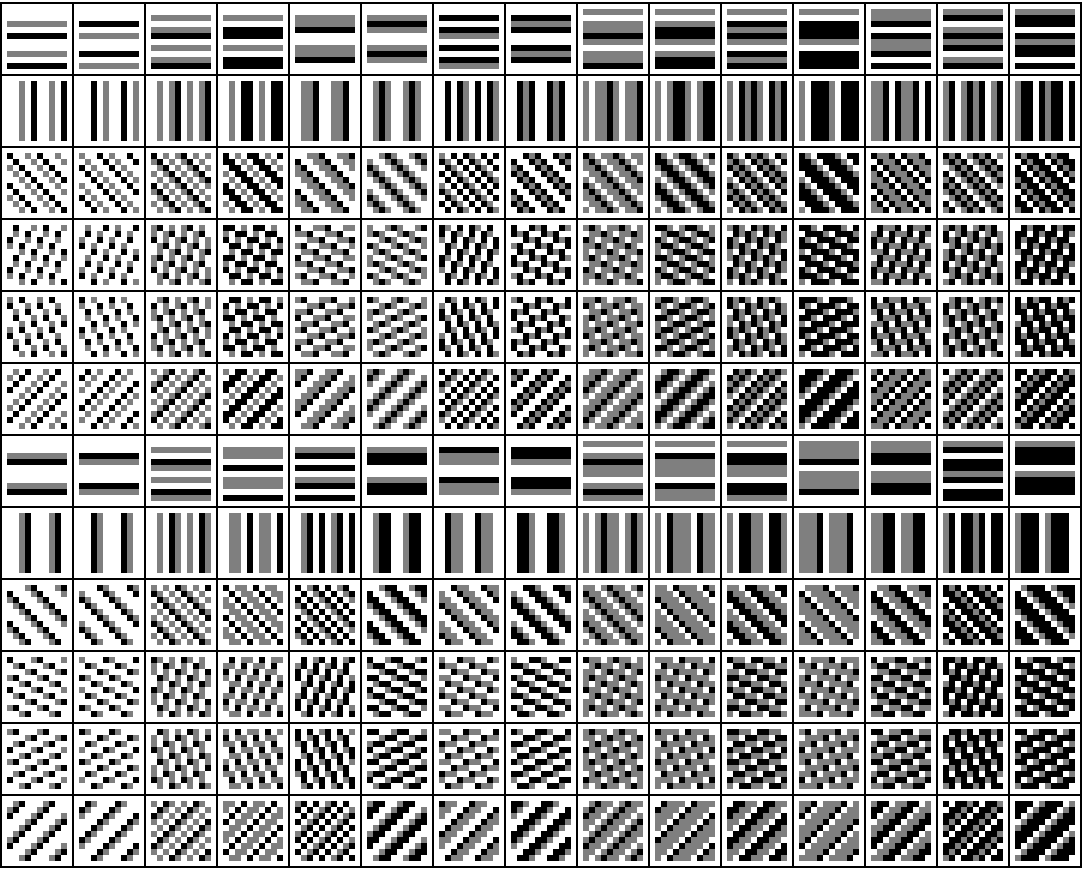Show samples of the 129064 order 16 binary patterns:

 In:=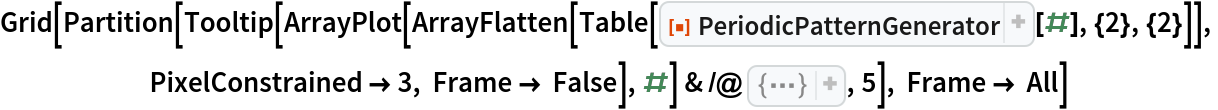Out=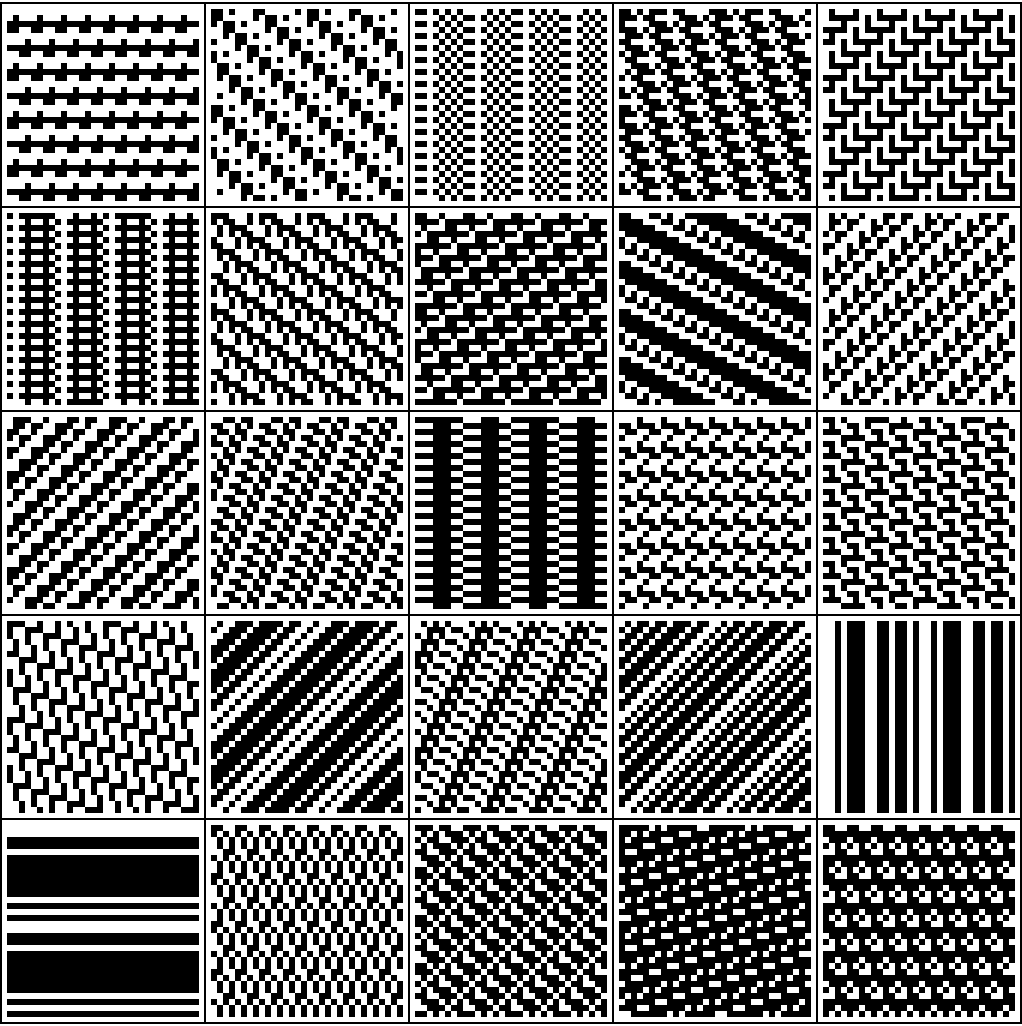## Version History

• 1.0.0 – 01 July 2022